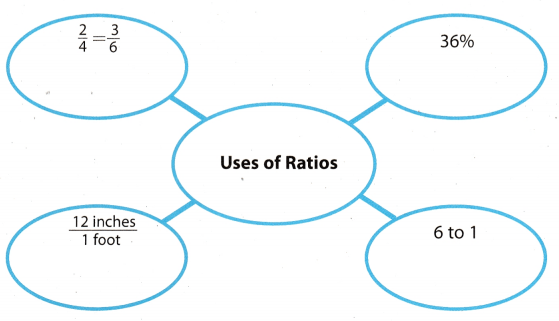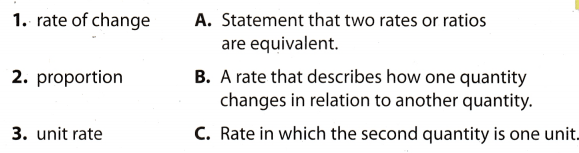# Texas Go Math Grade 7 Module 2 Answer Key Rates and Proportionality

Refer to our Texas Go Math Grade 7 Answer Key Pdf to score good marks in the exams. Test yourself by practicing the problems from Texas Go Math Grade 7 Module 2 Answer Key Rates and Proportionality.

## Texas Go Math Grade 7 Module 2 Answer Key Rates and Proportionality

Divide.

Question 1.
$$\frac{3}{4}$$ ÷ $$\frac{4}{5}$$ _____________
Multiply by the reciprocal of the divisor:
= $$\frac{3}{4}$$ × $$\frac{5}{4}$$
= $$\frac{15}{16}$$

Question 2.
$$\frac{5}{9}$$ ÷ $$\frac{10}{11}$$ _____________
Multiply by the reciprocal of the divisor:
= $$\frac{5}{9}$$ × $$\frac{11}{10}$$
= $$\frac{11}{18}$$

Question 3.
$$\frac{3}{8}$$ ÷ $$\frac{1}{2}$$ _____________
Multiply by the reciprocal of the divisor:
= $$\frac{3}{8}$$ × $$\frac{2}{1}$$
= $$\frac{3}{4}$$

Question 4.
$$\frac{16}{21}$$ ÷ $$\frac{8}{9}$$ _____________
Multiply by the reciprocal of the divisor:
= $$\frac{16}{21}$$ × $$\frac{9}{8}$$
= $$\frac{6}{7}$$

Write the ordered pair for each point.Question 5.
B ____________
B(-4, 1)

Question 6.
C ____________
C(3, 0)

Question 7.
D _____________
D(5, 4)

Question 8.
E _____________
E(-2, -2)

Question 9.
F _____________
F(0, 0)

Question 10.
G _____________
G(-4, 0)

Visualize Vocabulary

Use the ✓ words to complete the graphic. You can put more than one word in each bubble.Understand Vocabulary

Match the term on the left to the definition on the right.